# Right Triangles and Trigonometry

## Objective

Verify algebraically and find missing measures using the Law of Cosines.

## Common Core Standards

### Core Standards

?

• G.SRT.D.10 — Prove the Laws of Sines and Cosines and use them to solve problems.

## Criteria for Success

?

1. Identify a situation where the law of sines is not an effective method to solving a triangle.
2. Given the Law of Cosines describe the relationship to the Pythagorean theorem.
3. Describe when you would use the Pythagorean theorem rather than the Law of Cosines to solve a right triangle.
4. Verify the Law of Cosines algebraically and use this to solve triangles.
5. Identify when to use the Law of Sines, Law of Cosines, or area formula in terms of sine to solve triangles or find missing measures. Find missing measures in non-right triangles in non-contextual problems.

## Tips for Teachers

?

Anchor Problem #1 will require the teacher to do a significant amount of modeling to define and algebraically verify the Law of Cosines. Reference EngageNY, Geometry, Module 2, Lesson 32: Teacher Version for more guidance on this.

## Anchor Problems

?

### Problem 1

The Law of Cosines states that:

Given ${\triangle ABC}$,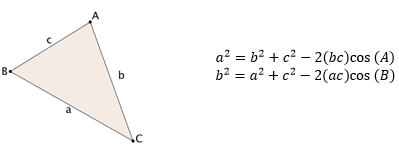What measurements of a triangle make the most sense to use the Law of Cosines?

### Problem 2

For each of the triangles below, would you use the Law of Sines, Law of Cosines, or Pythagorean theorem to find the value of $x$? Explain your reasoning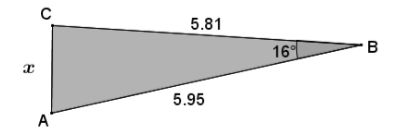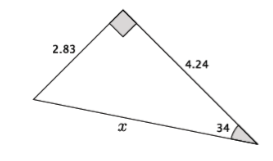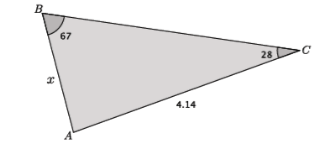#### References

EngageNY Mathematics Geometry > Module 2 > Topic E > Lesson 33Opening Exercise

Geometry > Module 2 > Topic E > Lesson 33 of the New York State Common Core Mathematics Curriculum from EngageNY and Great Minds. © 2015 Great Minds. Licensed by EngageNY of the New York State Education Department under the CC BY-NC-SA 3.0 US license. Accessed Dec. 2, 2016, 5:15 p.m..

Modified by Fishtank Learning, Inc.

## Problem Set

?

The following resources include problems and activities aligned to the objective of the lesson that can be used to create your own problem set.

• Include problems where students algebraically verify the Law of Cosines on their own.
• Include problems where students need to determine whether using the Law of Sines, Law of Cosines, Pythagorean theorem, or area formulas would be the most efficient way to find the missing measurements.

?

Given ${\triangle DEF}$, use the Law of Cosines to find the side length marked $d$ to the nearest tenth.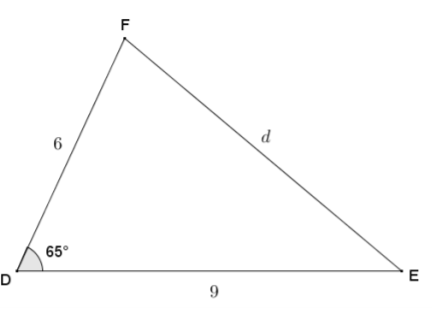#### References

EngageNY Mathematics Geometry > Module 2 > Topic E > Lesson 33Exit Ticket, Question #1

Geometry > Module 2 > Topic E > Lesson 33 of the New York State Common Core Mathematics Curriculum from EngageNY and Great Minds. © 2015 Great Minds. Licensed by EngageNY of the New York State Education Department under the CC BY-NC-SA 3.0 US license. Accessed Dec. 2, 2016, 5:15 p.m..

Modified by Fishtank Learning, Inc.